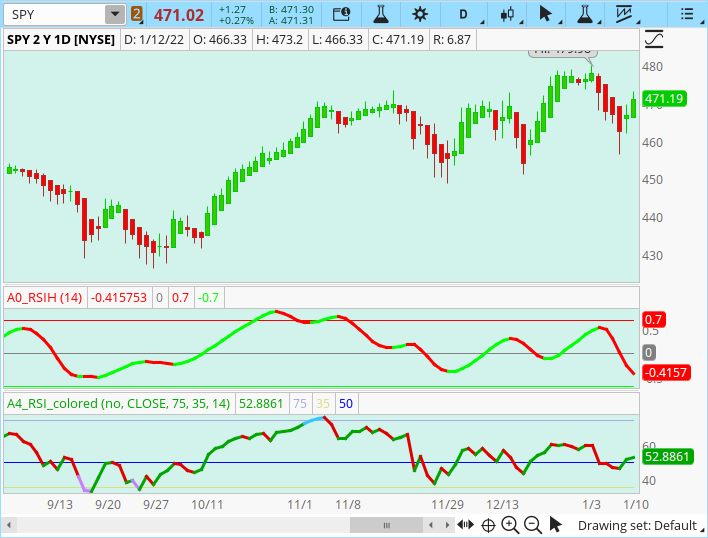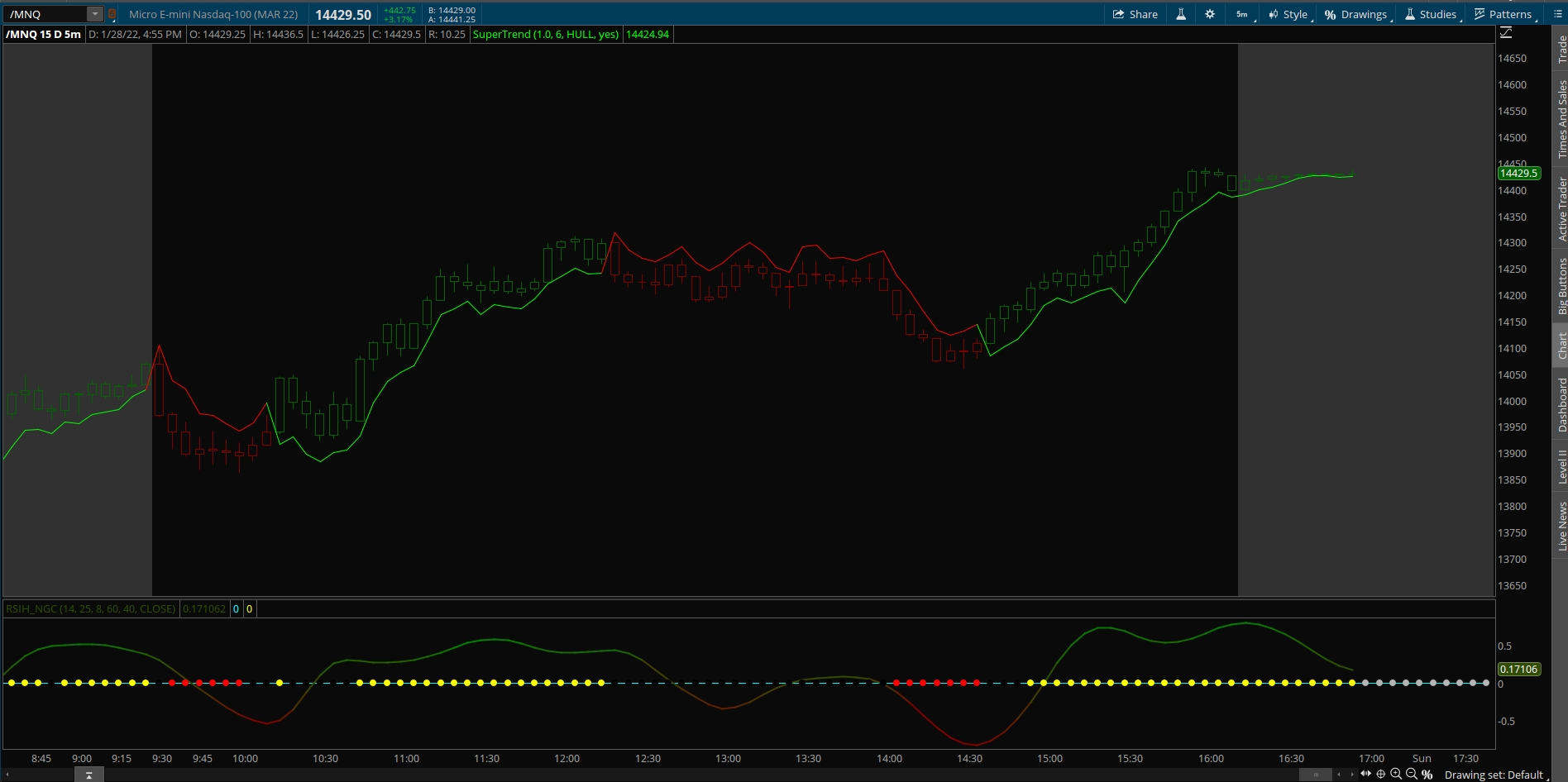# Ehler's (Yet Another) Improved RSI For ThinkOrSwim

#### MerryDay

Staff member
Staff
VIP
Ehler's (Yet Another) Improved RSI For ThinkOrSwim
The indicator is pictured in the 1st lower study with the ToS RSI in the 2nd lower chart for comparison.Ruby:
``````declare lower;

input length = 14;

def cu = fold i = 1 to length + 1 with up do up + if GetValue(close, i - 1, length - 1) > GetValue(close, i, length) then (1 - Cos(2 * Double.Pi * i / (length + 1 ))) * (GetValue(close, i - 1, length - 1) - GetValue(close, i, length)) else 0;
def cd = fold j = 1 to length + 1 with down do down + if GetValue(close, j, length) > GetValue(close, j - 1, length - 1) then (1 - Cos(2 * Double.Pi * j / (length + 1 ))) * (GetValue(close, j, length) - GetValue(close, j - 1, length - 1)) else 0;

plot RSIH = if (cu + cd) != 0 then (cu - cd) / (cu + cd) else 0;
plot ZeroLine = 0;
plot overbought = .70;
plot oversold = -.70 ;

ZeroLine.SetDefaultColor(GetColor(7));

# plots contributed by @bigboss
overbought.SetDefaultColor(COLOR.RED);
oversold.SetDefaultColor(COLOR.GREEN);
zeroline.SetDefaultColor(COLOR.GRAY);
RSIH.AssignValueColor(if RSIH < RSIH then COLOR.RED else if RSIH > RSIH then COLOR.GREEN else COLOR.WHITE);``````

Last edited:
declare lower;

input length = 14;

def cu = fold i = 1 to length + 1 with up do up + if GetValue(close, i - 1, length - 1) > GetValue(close, i, length) then (1 - Cos(2 * Double.Pi * i / (length + 1 ))) * (GetValue(close, i - 1, length - 1) - GetValue(close, i, length)) else 0;
def cd = fold j = 1 to length + 1 with down do down + if GetValue(close, j, length) > GetValue(close, j - 1, length - 1) then (1 - Cos(2 * Double.Pi * j / (length + 1 ))) * (GetValue(close, j, length) - GetValue(close, j - 1, length - 1)) else 0;

plot RSIH = if (cu + cd) != 0 then (cu - cd) / (cu + cd) else 0;
plot ZeroLine = 0;

RSIH.SetDefaultColor(GetColor(4));
ZeroLine.SetDefaultColor(GetColor(7));

I like a lot of Ehlers' indicators. I added RSI on the zeroline with OB OS of 60 40 and it's now a decent secondary confirmation indicator.
edit Mobius' Supertrend on chart
Code:
``````input RSI_Wilder_length = 8;
input RSI_Wilder_over_bought = 60;
input RSI_Wilder_over_sold = 40;
input RSI_Wilder_price_type = close;
def RSIplot = reference RSI(RSI_Wilder_length, RSI_Wilder_over_bought,
RSI_Wilder_over_sold,
RSI_Wilder_price_type);
plot RSI_line = 0;
RSI_line.SetPaintingStrategy(PaintingStrategy.POINTS);
Rsi_line.SetLineWeight(5);
RSI_line.AssignValueColor(if RSIplot >= RSI_Wilder_over_bought then CreateColor(255,255, 0) else if
RSIplot <=
RSI_Wilder_over_sold then CreateColor(255,0, 0) else CreateColor(0,0, 0));``````Last edited:
I like a lot of Ehlers' indicators. I added RSI on the zeroline with OB OS of 60 40 and it's now a decent secondary confirmation indicator.
edit Mobius' Supertrend on chart
Code:
``````input RSI_Wilder_length = 8;
input RSI_Wilder_over_bought = 60;
input RSI_Wilder_over_sold = 40;
input RSI_Wilder_price_type = close;
def RSIplot = reference RSI(RSI_Wilder_length, RSI_Wilder_over_bought,
RSI_Wilder_over_sold,
RSI_Wilder_price_type);
plot RSI_line = 0;
RSI_line.SetPaintingStrategy(PaintingStrategy.POINTS);
Rsi_line.SetLineWeight(5);
RSI_line.AssignValueColor(if RSIplot >= RSI_Wilder_over_bought then CreateColor(255,255, 0) else if
RSIplot <=
RSI_Wilder_over_sold then CreateColor(255,0, 0) else CreateColor(0,0, 0));``````

View attachment 13272
Interesting way to plot RSI. Thx!!

### Not the exact question you're looking for?

87k+ Posts
138 Online## The Market Trading Game Changer

Join 2,500+ subscribers inside the useThinkScript VIP Membership Club
• Exclusive indicators
• Proven strategies & setups
• Private Discord community
• Exclusive members-only content
• 1 full year of unlimited support

What is useThinkScript?

useThinkScript is the #1 community of stock market investors using indicators and other tools to power their trading strategies. Traders of all skill levels use our forums to learn about scripting and indicators, help each other, and discover new ways to gain an edge in the markets.

How do I get started?

We get it. Our forum can be intimidating, if not overwhelming. With thousands of topics, tens of thousands of posts, our community has created an incredibly deep knowledge base for stock traders. No one can ever exhaust every resource provided on our site.

If you are new, or just looking for guidance, here are some helpful links to get you started.

What are the benefits of VIP Membership?